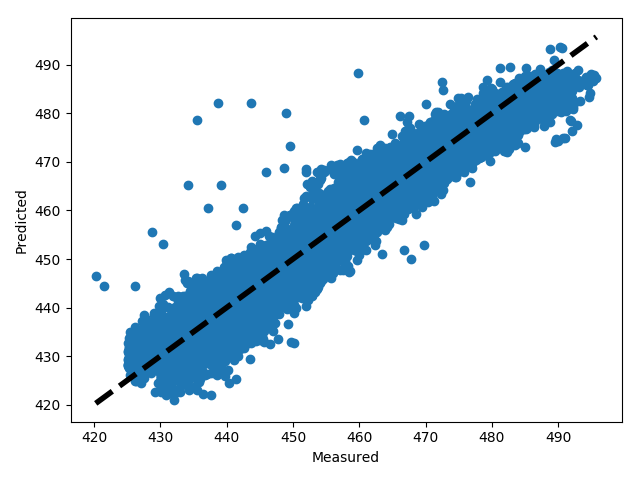1. 获取数据，定义问题

PE=θ0+θ1∗AT+θ2∗V+θ3∗AP+θ4∗RHPE=θ0+θ1∗AT+θ2∗V+θ3∗AP+θ4∗RH

3. 用pandas来读取数据

import matplotlib.pyplot as plt
%matplotlib inline
import numpy as np
import pandas as pd
from sklearn import datasets, linear_model

#读取前五行数据，如果是最后五行，用data.tail()

AT V AP RH PE
0 8.34 40.77 1010.84 90.01 480.48
1 23.64 58.49 1011.40 74.20 445.75
2 29.74 56.90 1007.15 41.91 438.76
3 19.07 49.69 1007.22 76.79 453.09
4 11.80 40.66 1017.13 97.20 464.43

4. 准备运行算法的数据

data.shape

X = data[['AT', 'V', 'AP', 'RH']]

AT V AP RH
0 8.34 40.77 1010.84 90.01
1 23.64 58.49 1011.40 74.20
2 29.74 56.90 1007.15 41.91
3 19.07 49.69 1007.22 76.79
4 11.80 40.66 1017.13 97.20

y = data[['PE']]

PE
0 480.48
1 445.75
2 438.76
3 453.09
4 464.43

5. 划分训练集和测试集

from sklearn.cross_validation import train_test_split
X_train, X_test, y_train, y_test = train_test_split(X, y, random_state=1)

print X_train.shape
print y_train.shape
print X_test.shape
print y_test.shape

(7176, 4)
(7176, 1)
(2392, 4)
(2392, 1)

可以看到75%的样本数据被作为训练集，25%的样本被作为测试集。

6. 运行scikit-learn的线性模型

终于到了临门一脚了，我们可以用scikit-learn的线性模型来拟合我们的问题了。scikit-learn的线性回归算法使用的是最小二乘法来实现的。代码如下：
from sklearn.linear_model import LinearRegression
linreg = LinearRegression()
linreg.fit(X_train, y_train)

print linreg.intercept_
print linreg.coef_

[ 447.06297099]
[[-1.97376045 -0.23229086  0.0693515  -0.15806957]]

PE=447.06297099−1.97376045∗AT−0.23229086∗V+0.0693515∗AP−0.15806957∗RHPE=447.06297099−1.97376045∗AT−0.23229086∗V+0.0693515∗AP−0.15806957∗RH

7. 模型评价#模型拟合测试集
y_pred = linreg.predict(X_test)
from sklearn import metrics
# 用scikit-learn计算MSE
print "MSE:",metrics.mean_squared_error(y_test, y_pred)
# 用scikit-learn计算RMSE
print "RMSE:",np.sqrt(metrics.mean_squared_error(y_test, y_pred))

MSE: 20.0804012021
RMSE: 4.48111606657

X = data[['AT', 'V', 'AP']]
y = data[['PE']]
X_train, X_test, y_train, y_test = train_test_split(X, y, random_state=1)
from sklearn.linear_model import LinearRegression
linreg = LinearRegression()
linreg.fit(X_train, y_train)
#模型拟合测试集
y_pred = linreg.predict(X_test)
from sklearn import metrics
# 用scikit-learn计算MSE
print "MSE:",metrics.mean_squared_error(y_test, y_pred)
# 用scikit-learn计算RMSE
print "RMSE:",np.sqrt(metrics.mean_squared_error(y_test, y_pred))

MSE: 23.2089074701
RMSE: 4.81756239919
可以看出，去掉RH后，模型拟合的没有加上RH的好，MSE变大了。

8. 交叉验证

我们可以通过交叉验证来持续优化模型，代码如下，我们采用10折交叉验证，即cross_val_predict中的cv参数为10：
X = data[['AT', 'V', 'AP', 'RH']]
y = data[['PE']]
from sklearn.model_selection import cross_val_predict
predicted = cross_val_predict(linreg, X, y, cv=10)
# 用scikit-learn计算MSE
print "MSE:",metrics.mean_squared_error(y, predicted)
# 用scikit-learn计算RMSE
print "RMSE:",np.sqrt(metrics.mean_squared_error(y, predicted))

MSE: 20.7955974619
RMSE: 4.56021901469

9. 画图观察结果

fig, ax = plt.subplots()
ax.scatter(y, predicted)
ax.plot([y.min(), y.max()], [y.min(), y.max()], 'k--', lw=4)
ax.set_xlabel('Measured')
ax.set_ylabel('Predicted')
plt.show()

10.完整代码：

import matplotlib.pyplot as plt
import pandas as pd
import numpy as np

#用pandas读取数据了
#准备样本特征X，用AT， V，AP和RH这4个列作为样本特征。
X = data[['AT', 'V', 'AP', 'RH']]
#准备样本输出y， 用PE作为样本输出。
y = data[['PE']]
#把X和y的样本组合划分成两部分，一部分是训练集，一部分是测试集
from sklearn.model_selection import train_test_split
X_train, X_test, y_train, y_test = train_test_split(X, y, random_state=1)
#查看下训练集和测试集的维度
print(X_train.shape)
print(y_train.shape)
print(X_test.shape)
print(y_test.shape)
#用scikit-learn的线性模型来拟合问题。scikit-learn的线性回归算法使用的是最小二乘法来实现的
from sklearn.linear_model import LinearRegression
linreg = LinearRegression()
linreg.fit(X_train, y_train)
LinearRegression(copy_X=True, fit_intercept=True, n_jobs=1, normalize=False)
#拟合完毕后，查看需要的模型系数结果：
print(linreg.intercept_)
print(linreg.coef_)
#模型拟合测试集
y_pred = linreg.predict(X_test)
#通过交叉验证来持续优化模型，采用10折交叉验证，即cross_val_predict中的cv参数为10：
from sklearn.model_selection import cross_val_predict
predicted = cross_val_predict(linreg, X, y, cv=10)
from sklearn import metrics
# 用scikit-learn计算MSE
print("MSE:",metrics.mean_squared_error(y_test, y_pred))
# 用scikit-learn计算RMSE
print("RMSE:",np.sqrt(metrics.mean_squared_error(y_test, y_pred)))
#画图观察结果
fig, ax = plt.subplots()
ax.scatter(y, predicted)
ax.plot([y.min(), y.max()], [y.min(), y.max()], 'k--', lw=4)
ax.set_xlabel('Measured')
ax.set_ylabel('Predicted')
plt.show()以上就是用scikit-learn和pandas学习线性回归的过程，希望可以对初学者有所帮助。